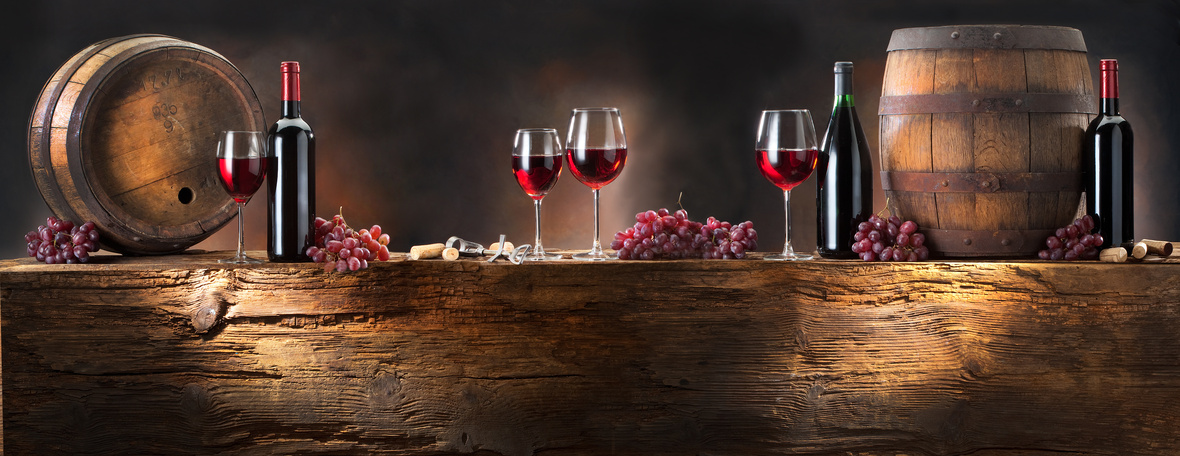# Imbibe for Causes and Level Up with SWW Sips

 /* styles */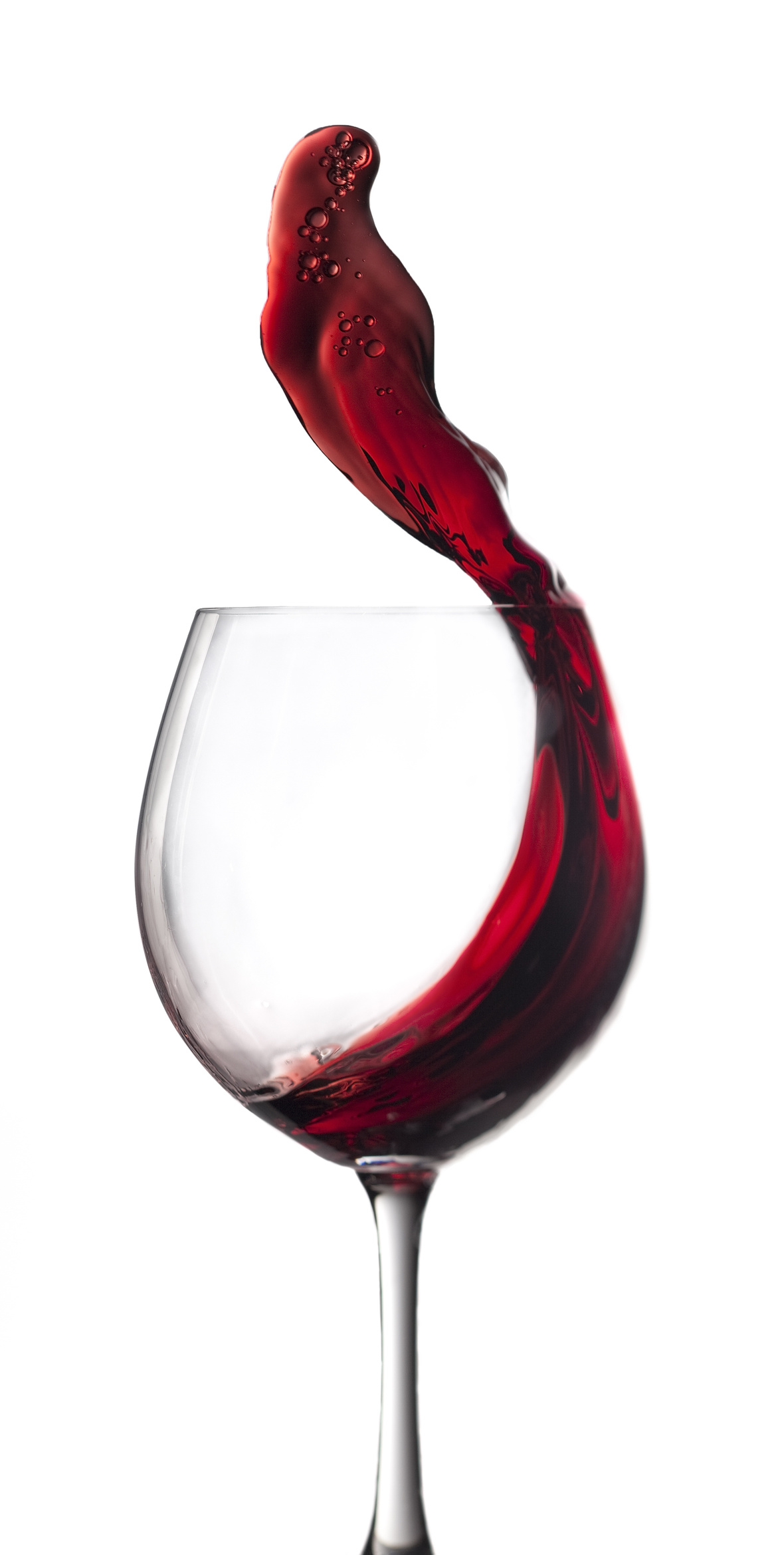Hi Wine & Spirits Lover,

The Shall We Wine team is excited to share our monthly newsletter. Our goal is to introduce you to our favorite selections, producers, distillers, varietals, regions and places to purchase and drink fabulous wine and spirits!

Cheers,

The Shall We Wine Team

 /* styles */
 table div table+table+table+table+table+table div table{width:100%;padding:0}table div table+table+table+table+table+table div table img{width:96.23%;padding:0;float:none}table div table+table+table+table+table+table div table td{width:100%;padding:0 1.88% 18px}/* styles */## BUBBLES, BOARDS, & BARRELHOUSE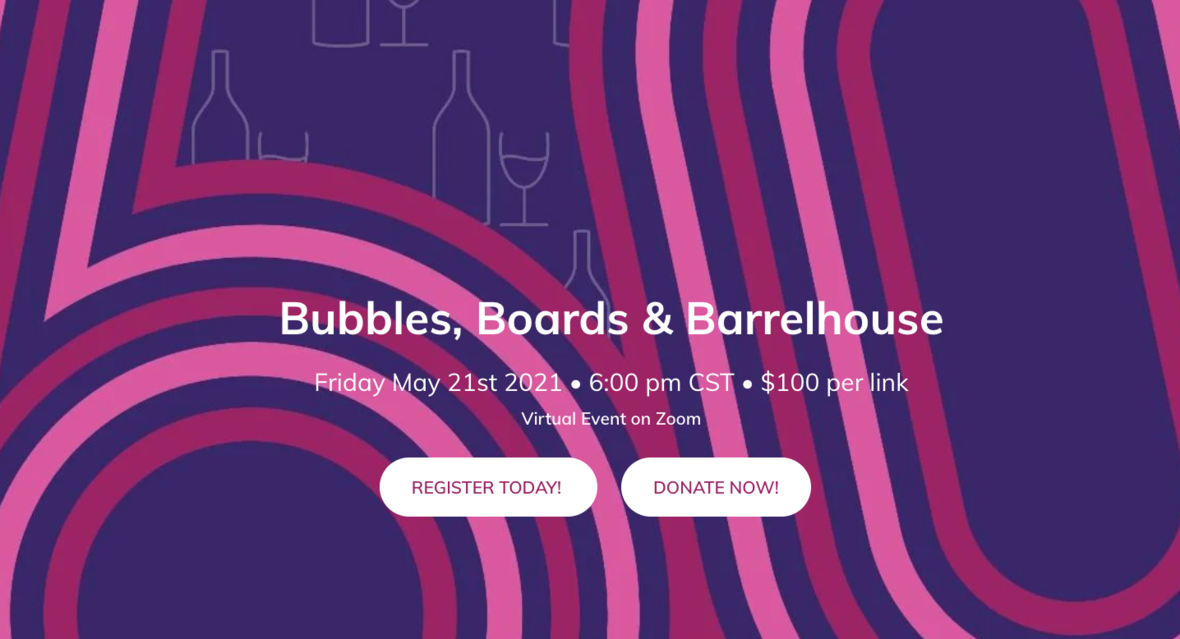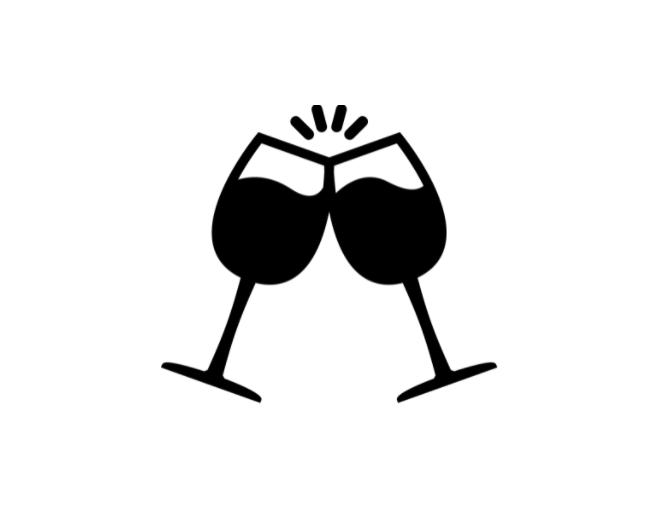Gather those in your Bubble for some Bubbles!

Join Shall We Wine for a virtual wine tasting hosted by the Urban Gateways Board of Directors on May 21, 2021 @ 6PM CST.

Plus, compete in the Charcuterie Board Contest to win a case of wine and a Grand Cru Membership to Shall We Wine (more than \$700 value!).

After the guided wine tasting, enjoy a virtual concert by MacArthur Genius and Urban Gateways alum Reginald Robinson, who will introduce you to the unique style of Barrelhouse music.

Make sure to order your wine by May 17 to ensure on-time delivery. Invite your friends to wine, dine, and dance in support of arts education for Chicago’s young people!

 table div table+table+table+table+table+table+table+table+table+table div table{width:100%;padding:0}table div table+table+table+table+table+table+table+table+table+table div table img{width:96.23%;padding:0;float:none}table div table+table+table+table+table+table+table+table+table+table div table td{width:100%;padding:0 1.88% 18px}/* styles *//* styles */

## DISCOVER WINES OF ARMENIAHave you tried wines from Armenia? Join Shall We Wine and Christopher Poldoian of Storica for a live tasting of Storica Wines from Armenia on June 11, 2021 @ 6PM CST for an Armenian wine primer and to see what makes the wines so special!

Storica was founded to share Armenia’s story as a burgeoning authority in wine and its history as the birthplace of wine.

 /* styles */
 /* styles */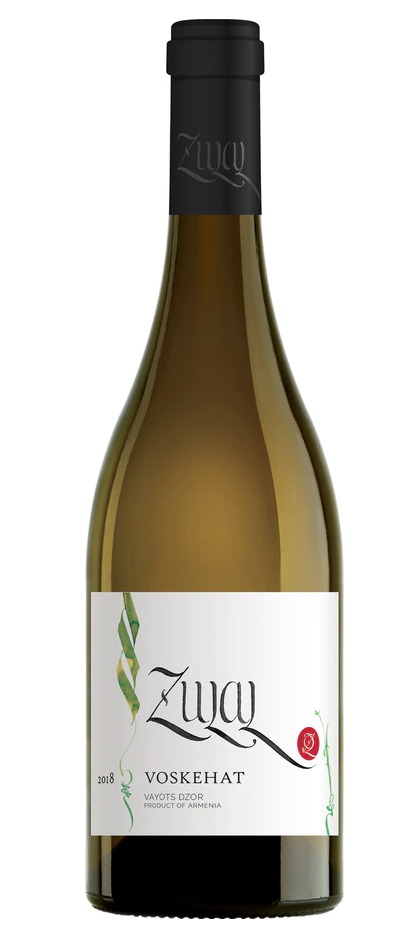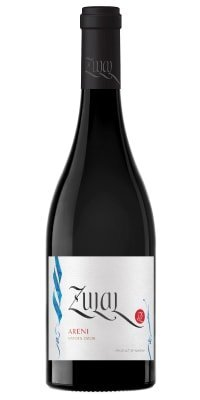/* styles */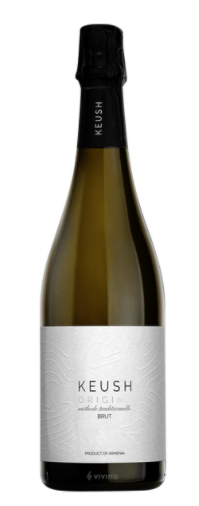/* styles */
 /* styles */
 /* styles */ Get free shipping with code: SWWFREESHIP Please order early to allow enough time for delivery! Can not deliver to: Alabama, Alaska, Arkansas, Delaware, Hawaii, Kentucky, Maine, Mississippi, Montana, New Hampshire, North Dakota, Oklahoma, Rhode Island, South Dakota, Utah, Vermont, West Virginia, and Wyoming
 table div table+table+table+table+table+table+table+table+table+table+table+table+table+table+table+table+table+table+table+table+table+table+table div table{width:100%;padding:0}table div table+table+table+table+table+table+table+table+table+table+table+table+table+table+table+table+table+table+table+table+table+table+table div table img{width:96.23%;padding:0;float:none}table div table+table+table+table+table+table+table+table+table+table+table+table+table+table+table+table+table+table+table+table+table+table+table div table td{width:100%;padding:0 1.88% 18px}/* styles */## SHALL WE WINE SIPS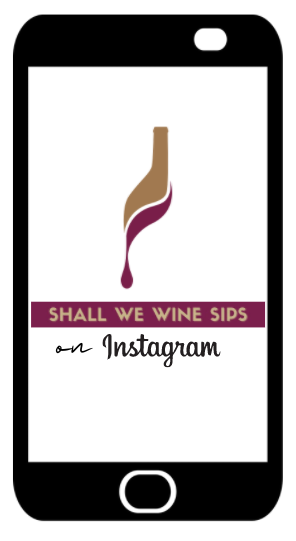/* styles */ Are you following Shall We Wine on IG? Level up your wine game and check out our weekly series, SHALL WE WINE SIPS Tuesdays at 6:00 PM CST where we interview some of our fave people in food and wine!
 table div table+table+table+table+table+table+table+table+table+table+table+table+table+table+table+table+table+table+table+table+table+table+table+table+table+table+table div table{width:100%;padding:0}table div table+table+table+table+table+table+table+table+table+table+table+table+table+table+table+table+table+table+table+table+table+table+table+table+table+table+table div table img{width:96.23%;padding:0;float:none}table div table+table+table+table+table+table+table+table+table+table+table+table+table+table+table+table+table+table+table+table+table+table+table+table+table+table+table div table td{width:100%;padding:0 1.88% 18px}/* styles */## 2021 WINE EDUCATION SERIES WITH SWW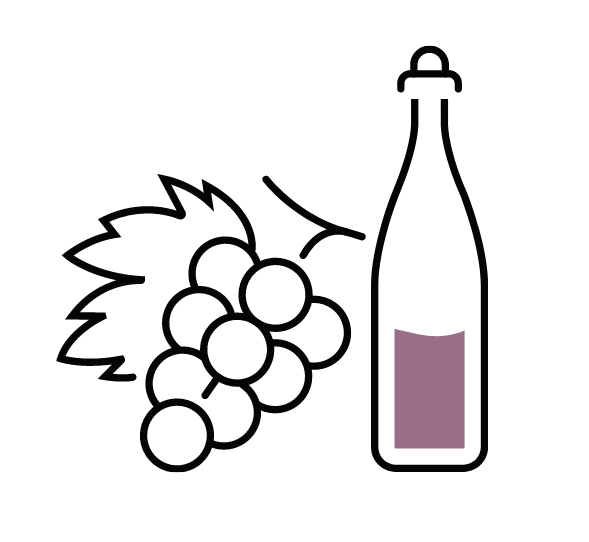Join Regine T. Rousseau, Founder and CEO for more of the amazing line-up of educational events in 2021!

See the list below, and click to reserve your spot.

Want to level up your wine drinking style without the extra fluff? Shall We Wine is a resource that helps translate complex terminology into language that individuals of every level of wine & spirits proficiency can appreciate… all while having fun!

The goal of these classes is to empower you to find the wines that fit your palate, the foods you enjoy and your mood. If you enjoy wine and are looking to deepen your knowledge of wine styles, grapes and regions, these are the classes for you!

 table div table+table+table+table+table+table+table+table+table+table+table+table+table+table+table+table+table+table+table+table+table+table+table+table+table+table+table+table+table+table div table{width:100%;padding:0}table div table+table+table+table+table+table+table+table+table+table+table+table+table+table+table+table+table+table+table+table+table+table+table+table+table+table+table+table+table+table div table img{width:96.23%;padding:0;float:none}table div table+table+table+table+table+table+table+table+table+table+table+table+table+table+table+table+table+table+table+table+table+table+table+table+table+table+table+table+table+table div table td{width:100%;padding:0 1.88% 18px}/* styles *//* styles */table div table+table+table+table+table+table+table+table+table+table+table+table+table+table+table+table+table+table+table+table+table+table+table+table+table+table+table+table+table+table+table+table+table div table{width:100%;padding:0}table div table+table+table+table+table+table+table+table+table+table+table+table+table+table+table+table+table+table+table+table+table+table+table+table+table+table+table+table+table+table+table+table+table div table img{width:96.23%;padding:0;float:none}table div table+table+table+table+table+table+table+table+table+table+table+table+table+table+table+table+table+table+table+table+table+table+table+table+table+table+table+table+table+table+table+table+table div table td{width:100%;padding:0 1.88% 18px}/* styles */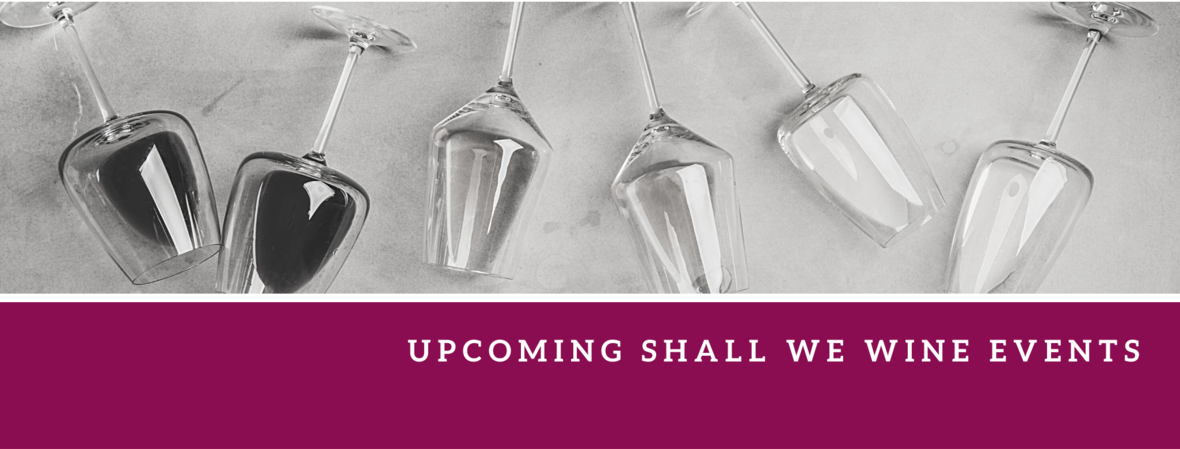/* styles */ Check out our complete events calendar to see what your next pour should be!table div table+table+table+table+table+table+table+table+table+table+table+table+table+table+table+table+table+table+table+table+table+table+table+table+table+table+table+table+table+table+table+table+table+table+table+table+table+table+table div table{width:100%;padding:0}table div table+table+table+table+table+table+table+table+table+table+table+table+table+table+table+table+table+table+table+table+table+table+table+table+table+table+table+table+table+table+table+table+table+table+table+table+table+table+table div table img{width:96.23%;padding:0;float:none}table div table+table+table+table+table+table+table+table+table+table+table+table+table+table+table+table+table+table+table+table+table+table+table+table+table+table+table+table+table+table+table+table+table+table+table+table+table+table+table div table td{width:100%;padding:0 1.88% 18px}/* styles */## AAPI HERITAGE MONTH

 /* styles */ This month we pay tribute to the generations of Asian and Pacific Islanders who have enriched America's history. While there are countless ways to support their community, below is a small glimpse of highlights in the beverage, food and media spaces: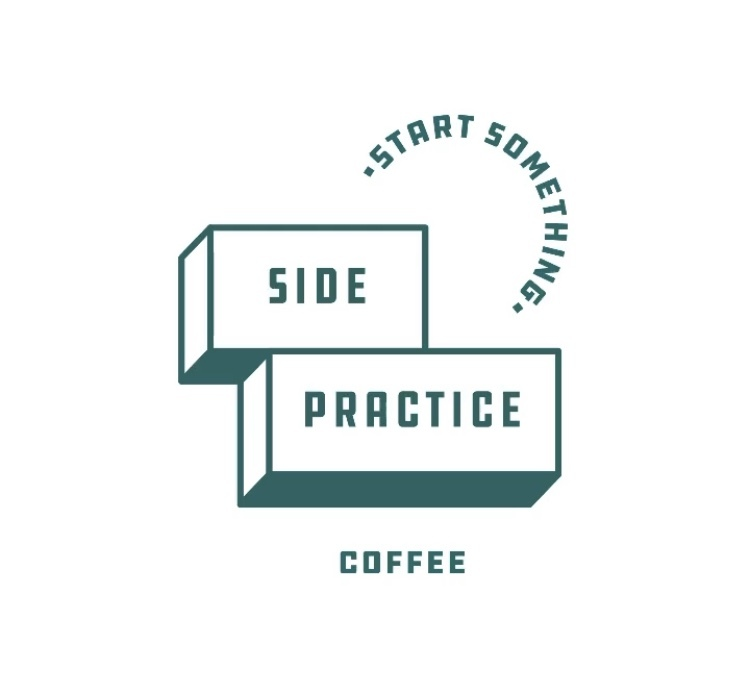The hours of Side Practice Coffee might be limited, but they work beyond the traditional thought of a local coffee shop. It's much more than that. Side Practice is an AAPI-owned business which showcases your side hustle, your side practice, in any medium. They have rotating features of artists and entrepreneurs from Chicagoland. This month alone has highlighted events like a Spring Swap to bring attention to sustainability, vinyl record level ups with South Rhodes, and a special COURSEWORK t-shirt collab in which all proceeds go toward #stopasianhate. Click here for more AAPI-Owned Businesses.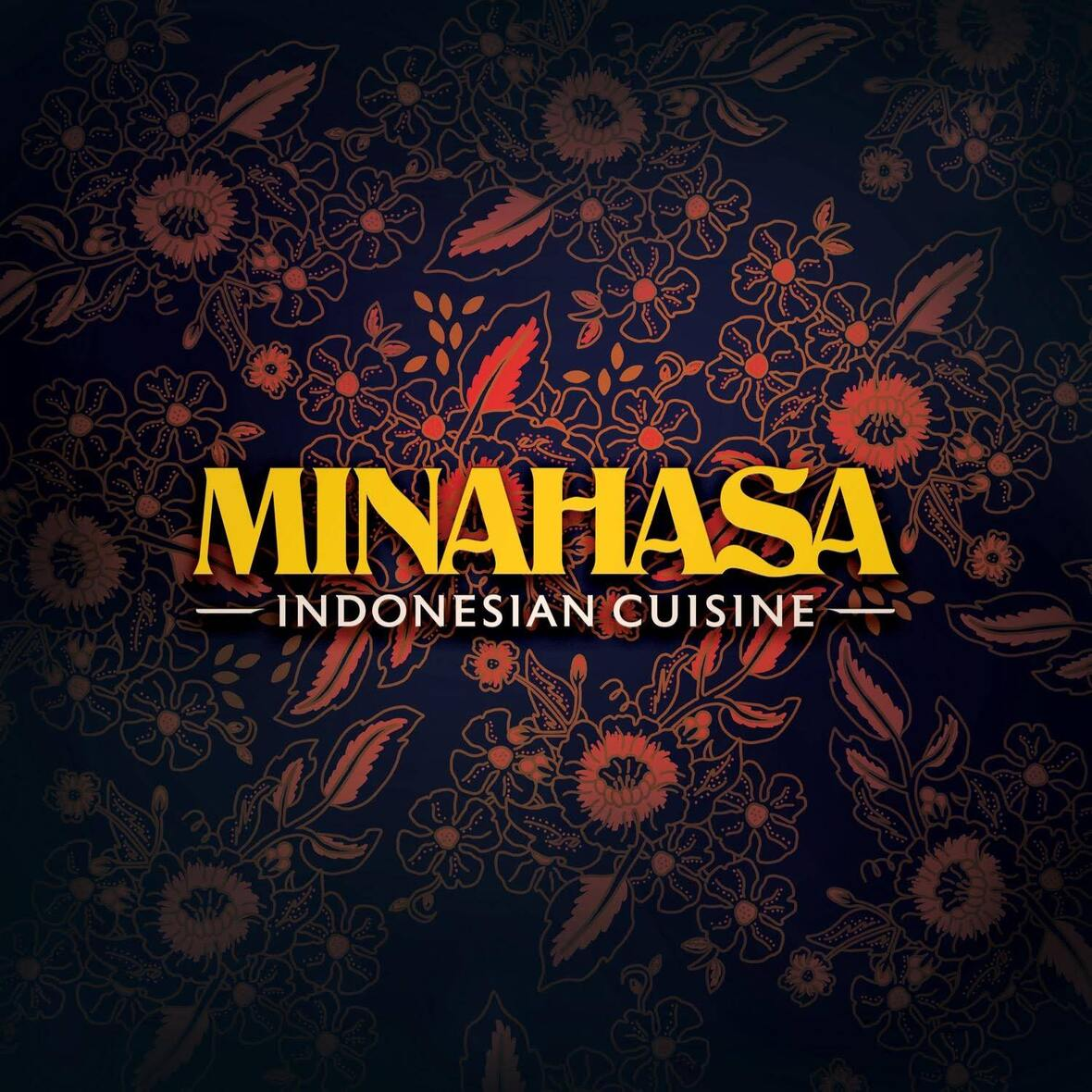Minahasa is one of the newer offerings of Revival Food Hall. Not only is it a great addition to the hall itself, but is also a sign of widening and progress in the small representation of Indonesian cuisine and its expansion. Special focus is given to regional offerings from Tomohon, Indonesia (in the mountains of North Sulawesi), along with selections inspired by Java and Sumatra, too.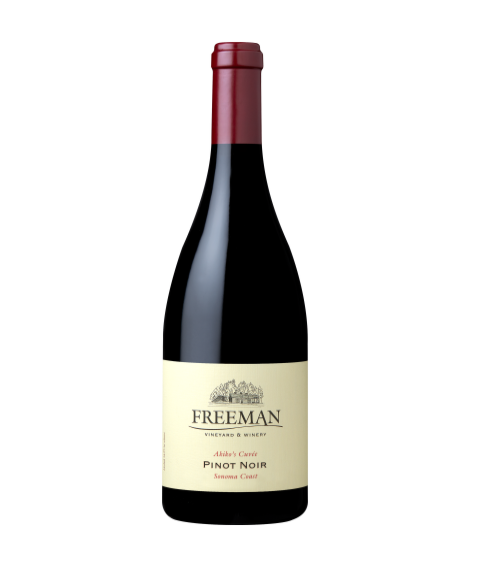Every year since their inaugural '02 vintage, Freeman has released Akiko’s Cuvée Pinot Noir. Akiko Freeman is 1/2 of the dynamic duo of of Freeman Winery in Sebastopol. She is native to Tokyo and marks her love of vino to her grandfather. "Akiko oversees every aspect of Freeman’s winemaking program, from the organic farming of the estate vineyards and the selection of grower partners to fermentations, racking, lot evaluations and blending." Freeman Winery is known for exceptional cool-climate Pinot Noir and Chardonnay. They also join the list of wineries which have been served at White House dinners. Former President Barack Obama and First Lady Michelle Obama hosted Japanese Prime Minister Shinzo Abe with Freeman Ryo-fu Chardonnay.VISIBLE Magazine is an online publication committed to making storytelling accessible and inclusive, focusing on the work of those whose voices have been intentionally ignored or suppressed by traditional media outlets. Founder Stephanie Drenka is DePaul University alum, who is currently based in Dallas and an Activist Writer & Adoptee Advocate. As a Korean adoptee herself, she also has a compelling assessment of the adoption process, especially those involving Asian children. She is the Dallas Truth, Racial Healing & Transformation Communications Director.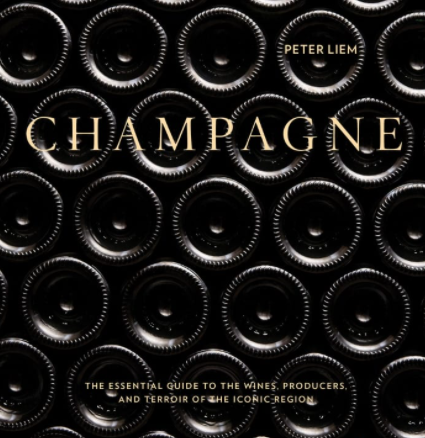Champagne geeks, unite! Peter Liem started out with ChampagneGuide.net, yet it evolved to so much more. This is to cheers the awesomeness that is Champagne: The Essential Guide to the Wines, Producers, and Terroirs of the Iconic Region (Boxed Book & Map Set). This really is more of a reference book, but it’s totally a different experience actually reading this book cover-to-cover. Try it! From the maps to in-depth producer profiles, this is the most comprehensive Champagne resource out there.

 table div table+table+table+table+table+table+table+table+table+table+table+table+table+table+table+table+table+table+table+table+table+table+table+table+table+table+table+table+table+table+table+table+table+table+table+table+table+table+table+table+table+table+table+table+table+table+table div table{width:100%;padding:0}table div table+table+table+table+table+table+table+table+table+table+table+table+table+table+table+table+table+table+table+table+table+table+table+table+table+table+table+table+table+table+table+table+table+table+table+table+table+table+table+table+table+table+table+table+table+table+table div table img{width:96.23%;padding:0;float:none}table div table+table+table+table+table+table+table+table+table+table+table+table+table+table+table+table+table+table+table+table+table+table+table+table+table+table+table+table+table+table+table+table+table+table+table+table+table+table+table+table+table+table+table+table+table+table+table div table td{width:100%;padding:0 1.88% 18px}/* styles */## DRINK WELL, DO GOOD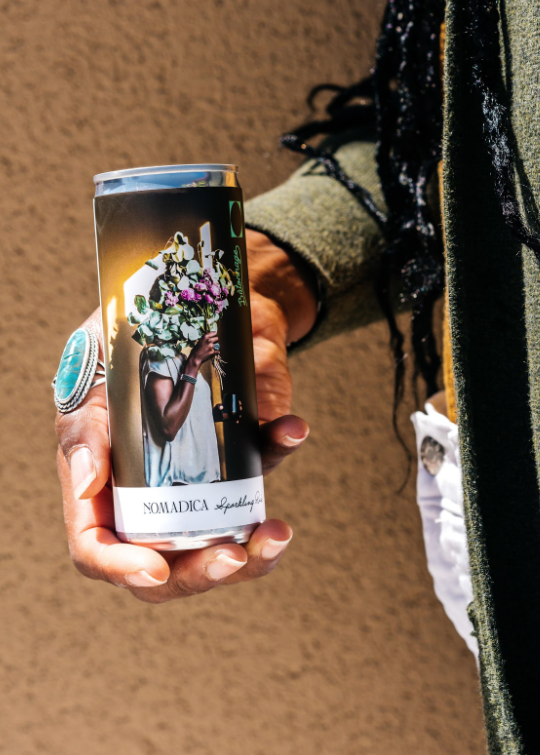BUY THE CAN, SUPPORT THE CAUSE

Cheers to Team Bâtonnage's exciting collaboration with Nomadica Wine. Nomadica is a female and LGBTQ+ owned wine brand. The collab helps raise funds for the Bâtonnage Mentorship Program! 100% of all proceeds from these 2018 Sparkling Rosé cans will go towards fostering a positive and welcoming wine industry community. Label artwork by the Alisha Sommer, Photo credit Emma K. Morris.

Interested in contributing to the ongoing success of Bâtonnage—the community, the annual Forum, and the newly launched Mentorship Program? Learn more about Bâtonnage: Women In Wine here.

 table div table+table+table+table+table+table+table+table+table+table+table+table+table+table+table+table+table+table+table+table+table+table+table+table+table+table+table+table+table+table+table+table+table+table+table+table+table+table+table+table+table+table+table+table+table+table+table+table+table+table div table{width:100%;padding:0}table div table+table+table+table+table+table+table+table+table+table+table+table+table+table+table+table+table+table+table+table+table+table+table+table+table+table+table+table+table+table+table+table+table+table+table+table+table+table+table+table+table+table+table+table+table+table+table+table+table+table div table img{width:96.23%;padding:0;float:none}table div table+table+table+table+table+table+table+table+table+table+table+table+table+table+table+table+table+table+table+table+table+table+table+table+table+table+table+table+table+table+table+table+table+table+table+table+table+table+table+table+table+table+table+table+table+table+table+table+table+table div table td{width:100%;padding:0 1.88% 18px}/* styles */## BLOG CENTRALWe have a blog! Here you will find things like our Wine of the Week and other special wine & spirits topics! Stay in the know and read up on some of the cool things we've been sharing at Shall We Wine.

 table div table+table+table+table+table+table+table+table+table+table+table+table+table+table+table+table+table+table+table+table+table+table+table+table+table+table+table+table+table+table+table+table+table+table+table+table+table+table+table+table+table+table+table+table+table+table+table+table+table+table+table+table+table div table{width:100%;padding:0}table div table+table+table+table+table+table+table+table+table+table+table+table+table+table+table+table+table+table+table+table+table+table+table+table+table+table+table+table+table+table+table+table+table+table+table+table+table+table+table+table+table+table+table+table+table+table+table+table+table+table+table+table+table div table img{width:96.23%;padding:0;float:none}table div table+table+table+table+table+table+table+table+table+table+table+table+table+table+table+table+table+table+table+table+table+table+table+table+table+table+table+table+table+table+table+table+table+table+table+table+table+table+table+table+table+table+table+table+table+table+table+table+table+table+table+table+table div table td{width:100%;padding:0 1.88% 18px}/* styles */## ENGAGING READS FOR THE MONTH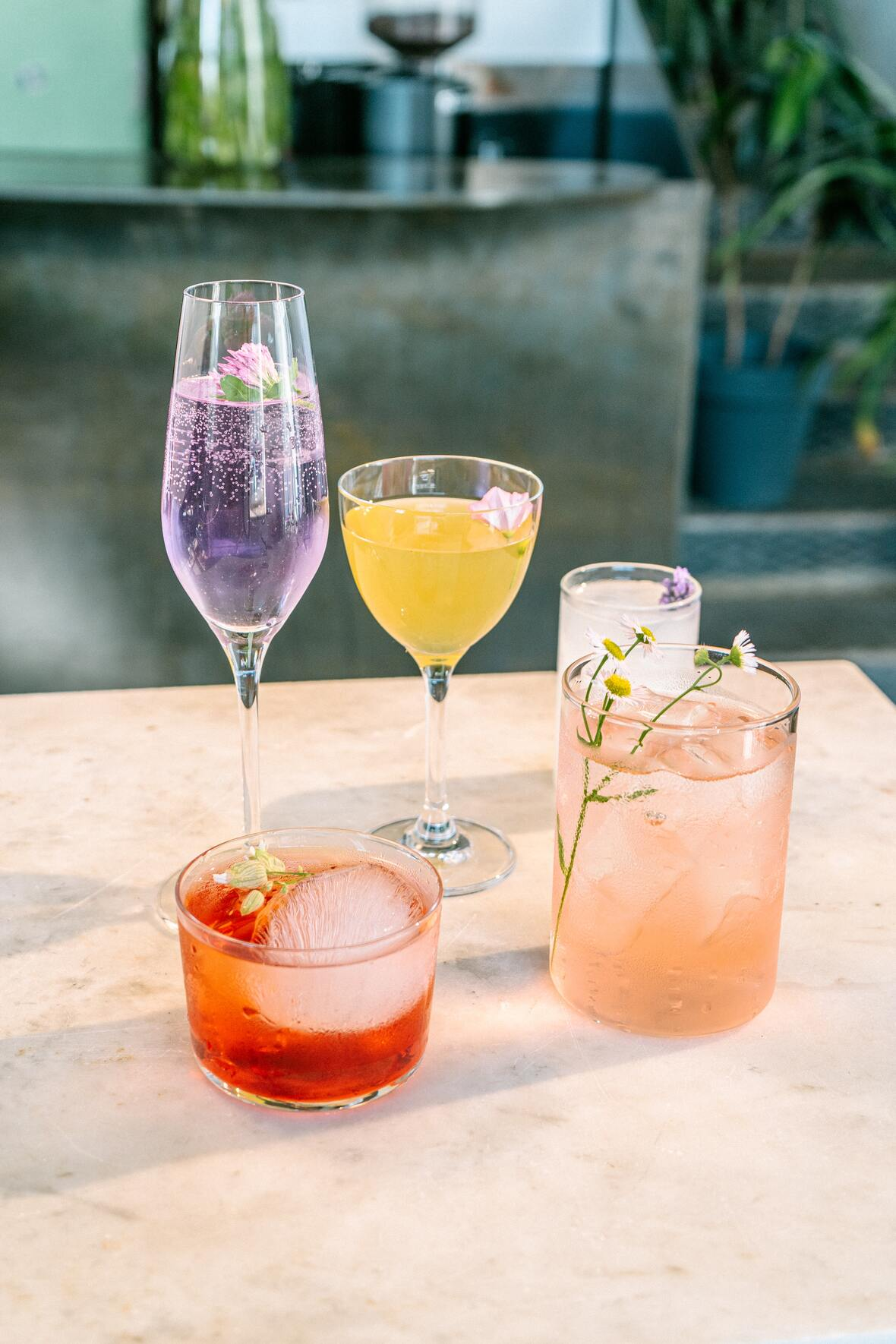Coconut Wine as a Symbol of Pride and Resistance
Wine Enthusiast

How Immigration and Diversity are Affecting the Future of the Restaurant Industry
Full Service Restaurant

Can Alcohol Be Composted?
VinePair

 table div table+table+table+table+table+table+table+table+table+table+table+table+table+table+table+table+table+table+table+table+table+table+table+table+table+table+table+table+table+table+table+table+table+table+table+table+table+table+table+table+table+table+table+table+table+table+table+table+table+table+table+table+table+table+table+table div table{width:100%;padding:0}table div table+table+table+table+table+table+table+table+table+table+table+table+table+table+table+table+table+table+table+table+table+table+table+table+table+table+table+table+table+table+table+table+table+table+table+table+table+table+table+table+table+table+table+table+table+table+table+table+table+table+table+table+table+table+table+table div table img{width:96.23%;padding:0;float:none}table div table+table+table+table+table+table+table+table+table+table+table+table+table+table+table+table+table+table+table+table+table+table+table+table+table+table+table+table+table+table+table+table+table+table+table+table+table+table+table+table+table+table+table+table+table+table+table+table+table+table+table+table+table+table+table+table div table td{width:100%;padding:0 1.88% 18px}/* styles */## STAY CONNECTED

 /* styles */ To stay in the know with the latest and greatest, make sure to follow us! Links are below.
 table div table+table+table+table+table+table+table+table+table+table+table+table+table+table+table+table+table+table+table+table+table+table+table+table+table+table+table+table+table+table+table+table+table+table+table+table+table+table+table+table+table+table+table+table+table+table+table+table+table+table+table+table+table+table+table+table+table+table+table div table{width:100%;padding:0}table div table+table+table+table+table+table+table+table+table+table+table+table+table+table+table+table+table+table+table+table+table+table+table+table+table+table+table+table+table+table+table+table+table+table+table+table+table+table+table+table+table+table+table+table+table+table+table+table+table+table+table+table+table+table+table+table+table+table+table div table table{padding:0;float:left!important;width:26.059%!important}table div table+table+table+table+table+table+table+table+table+table+table+table+table+table+table+table+table+table+table+table+table+table+table+table+table+table+table+table+table+table+table+table+table+table+table+table+table+table+table+table+table+table+table+table+table+table+table+table+table+table+table+table+table+table+table+table+table+table+table div table td{padding-left:29px;padding-right:29px}table div table+table+table+table+table+table+table+table+table+table+table+table+table+table+table+table+table+table+table+table+table+table+table+table+table+table+table+table+table+table+table+table+table+table+table+table+table+table+table+table+table+table+table+table+table+table+table+table+table+table+table+table+table+table+table+table+table+table+table div table table+table,table div table+table+table+table+table+table+table+table+table+table+table+table+table+table+table+table+table+table+table+table+table+table+table+table+table+table+table+table+table+table+table+table+table+table+table+table+table+table+table+table+table+table+table+table+table+table+table+table+table+table+table+table+table+table+table+table+table+table+table div table table+table+table{float:left!important;width:26.059%!important}table div table+table+table+table+table+table+table+table+table+table+table+table+table+table+table+table+table+table+table+table+table+table+table+table+table+table+table+table+table+table+table+table+table+table+table+table+table+table+table+table+table+table+table+table+table+table+table+table+table+table+table+table+table+table+table+table+table+table+table div table table td,table div table+table+table+table+table+table+table+table+table+table+table+table+table+table+table+table+table+table+table+table+table+table+table+table+table+table+table+table+table+table+table+table+table+table+table+table+table+table+table+table+table+table+table+table+table+table+table+table+table+table+table+table+table+table+table+table+table+table+table div table table+table td,table div table+table+table+table+table+table+table+table+table+table+table+table+table+table+table+table+table+table+table+table+table+table+table+table+table+table+table+table+table+table+table+table+table+table+table+table+table+table+table+table+table+table+table+table+table+table+table+table+table+table+table+table+table+table+table+table+table+table+table div table table+table+table td{padding-left:0;padding-right:20px}table div table+table+table+table+table+table+table+table+table+table+table+table+table+table+table+table+table+table+table+table+table+table+table+table+table+table+table+table+table+table+table+table+table+table+table+table+table+table+table+table+table+table+table+table+table+table+table+table+table+table+table+table+table+table+table+table+table+table+table div table table+table+table+table{float:left!important;width:21.822999999999993%!important}table div table+table+table+table+table+table+table+table+table+table+table+table+table+table+table+table+table+table+table+table+table+table+table+table+table+table+table+table+table+table+table+table+table+table+table+table+table+table+table+table+table+table+table+table+table+table+table+table+table+table+table+table+table+table+table+table+table+table+table div table table+table+table+table td{padding-left:0;padding-right:0}/* styles *//* styles */ Learn, Drink, Repeat! The Shall We Wine Team
 Like   Tweet   Pin   +1   in